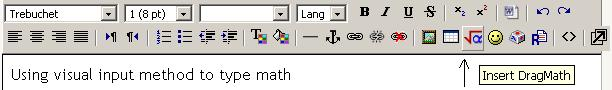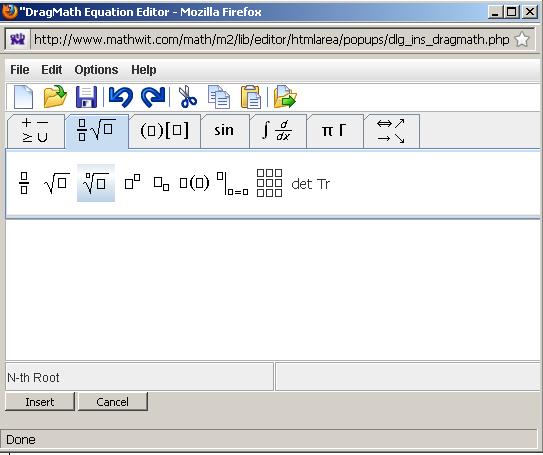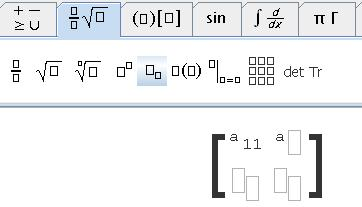## Using visual input method to type math symbols and equations

A few external free resources for tying math online and visualization:

LaTeX Base: https://latexbase.com/

ShareLaTeX(now part of Overleaf)https://www.sharelatex.com/

It is very useful to learn how to input some math by writing some latex code. Most of the science books, articles and papers are written by using latex or using visual aids to input latex code, and in some cases, the latex code is converted directly into symbols, equations, and pictures. In this article, you will quickly learn how to input some simple math and possibly learn some simple latex markup (know HTML?). A well know latex mark up for power is ^, for example, 2^3=8 ($$2^3=8$$). So you already know something!

You can use visual input method by clicking the $$\sqrt\alpha$$ icon near the smiley face of the toolbar to generate code. See the following picture.It will take a few seconds for the pop-up window to load its contents, but after that, the next time it will load much faster. Then, you can click the tabs to find symbols and types you need, it will convert the symbols into latex code. The following picture shows how to type nth root. The buttons are darker to indicate that they are selected.After you click the nth root symbol, you can input numbers below, for example, the following picture show you inputted $$\sqrt{27}$$. But you still want to input an equal sign and the answer. How to do that?

Click the button labeled with $$\begin{array}{cc} + & -\\\\ \geq & \cup \end{array}$$ to select equal sign and drag it to the right of the 3rd root of 27 and then type your answer. The last step is to click the insert button located at the lower left corner.After you click the insert button, the pop-up window will disappear and the code \$\$ \sqrt{27} =3\$\$ will be inserted into the text area and after you submit, it will be the following

$$\sqrt{27} =3.$$

Notice that the expression will start in a new line. If double dollar sign (\$\$) enclosed expressions are displayed in a new line and aligned left, to make an expression inline, you can change the double dollar signs to single dollar signs, for example, \$\$\sqrt{27} =3\$\$ gives you $$\sqrt{27} =3$$.

For long formula, you can enclose it inand. For example,\sqrt{27} =3 \Rightarrow 3^3=27gives
$$\sqrt{27} =3 \Rightarrow 3^3=27.$$

The effect is making it in a new line and centered.

Next you can practice to type a 2 by 2 matrix $$\left( \begin{array}{ccc} a_{11} & a_{12}\\\\ a_{21} & a_{22} \end{array} \right)$$.

You need to select the right tabs, after you click the matrix tab which consists of 9 small squares in 3 rows and 3 columns, you will see the following picture.The next step, type a's with subscripts by clicking the subscript icon and drag it in the four placeholders in the matrix. Finally, you can type the entries of the matrix as in the following picture.After you are done, insert the code and save.

If you get some red error messages, you possibly need to leave a space between \\ and $$a_{21}$$ (a matrix entry, the first element of the second row). If the matrix only displays in one line like an array, you need to escape \ by \\, change double backward slashes \\ to \\\\ , i.e., 4 backward slashes.